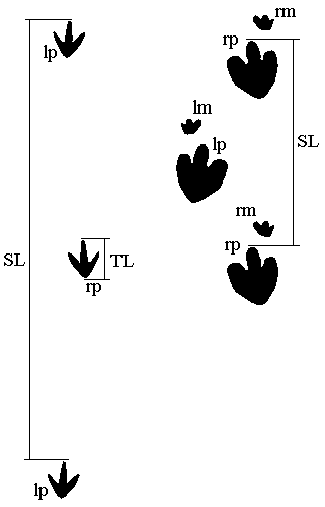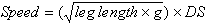# EARTH SCIENCE LAB Dinosaur Trackways

### Goal

To become familiar with dinosaur trackways and speeds.

Dinosaur remains have been discovered on every continent. In North America dinosaurs are known from such places as Alabama, Alaska, New Jersey, Alberta, Colorado, Montana, Utah and Wyoming. Some of the most common dinosaur fossils are footprints and trackways. Trackways give scientists some of the only direct evidence of dinosaur behavior. Based on trackways and track morphology, inferences about dinosaur size, gait, activity and speed of movement can be made. To interpret these trackways, however, modern organisms must be studied to determine what behavior leads to the formation of certain kinds of tracks. Imagine the different kinds of tracks you could make in various substrates (soft mud, wet sand, dry sand and so forth) or in various activities (running, walking, or jumping). Each behavior in each kind of substrate will leave a different kind of track.

The morphology and general behavior of an organism can also be determined by the trackway. The number and pairing of tracks indicate whether the creature was bipedal (walked on two feet) or quadruped (walked on four feet). In addition, the shape of the foot is an indication of behavior. Relatively narrow feet that have pointed toes are typical of carnivorous dinosaurs - all of which were bipedal. Broad feet with rounded toes are typical of herbivores - typically larger animals that require greater areas for support. Some ornithopods had smaller feet with a hoof-like pad.

Assuming that most dinosaur trackways represent normal movement (typical gait) the size of the dinosaur and its speed can be estimated by the tracks and the length of its stride.

### Size of Dinosaurs Based on Tracks

The true size of a dinosaur is best measured from preserved bones. Based on comparative anatomy, a single bone can give a rough indication of the size of the organism. By empirical study (measurement of many organisms) it has been found that most dinosaurs hind feet are one quarter (1/4) of the leg length. Based on the length of the leg, the overall length of the trackmaker can be determined by comparison to models of similar species. Well preserved tracks are needed for this kind of estimate.

### Assignment

Complete the following steps each of the dinosaur trackways listed. All measurements are in meters (m). Actual speed will be reported in meters per second (m/s) and miles per hour (mph). Record the information for this exercise in the table below. Figure 1 shows how the various measurements are made on fossil trackways.Figure 1. Measurement of track length (TL) and stride length (SL) from a stylized carnivorous dinosaur (theropod) trackway (A) and stylized quadrupedal herbivorous (ornithopod) trackway (B). Tracks are labeled right (r), left (l), manus (front) (m), and pes (hind) (p). (diagram modified from Over, 1995)

1. Calculate the Leg Length.

2. Calculate the Relative Stride Length. Relative Stride Length is equal to the stride length divided by the leg length.

3. The Dimensionless Speed is based on the fact that upright tetrapods (humans are bipedal tetrapods), regardless of size, move in the same way. Dimensionless speed is determined by the formula:g = acceleration due to gravity (9.8 m/s2)

This is easily determined for various animals. When dimensionless speed data are plotted on a graph versus relative stride length the regression line can be used to determine the dimensionless speed of dinosaurs based on trackway measurements of leg length and stride length. The above equation is impossible for us to use in this case due to the fact that the speed is not known. In order to calculate the Dimensionless Speed use the following equations, where RSL is Relative Stride Length, and DS is Dimensionless Speed:

RSL = 1.1 x DS + 1
DS = (RSL - 1)/1.1

4. The actual speed in meters per second is calculated using the following formula:5. Convert m/s (meters per second) to mph (miles per hour). To do this, first convert meters to miles. Then multiply by 60; this will give you miles per minute. Then multiply by 60 again; this will give you miles per hour.

NOTE: Calculate and round all values to 2 decimal places. Values less than 1 must have a 0 in front of the decimal - 0.32, 0.67, etc.

# Dinosaur Foot Length (m) Stride Length (m) Leg Length (m) Relative Stride Length Dimensionless Speed Actual Speed (m/s) Actual Speed (mph)
Ex. Hadrosaur 0.3 1.4 1.2 1.17 0.15 0.51 1.15
1 Struthiomimus 0.23 1.34
2 Velociraptor 0.25 2.72
3 Euoplocephalus 0.40 1.87
4 Stegosaurus 0.35 1.90

(This lab has been adapted and modified from Over, D. Jeffery, 1995, Determining dinosaur speed. Journal of Geological Education, v. 43, p. 207-211.)